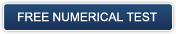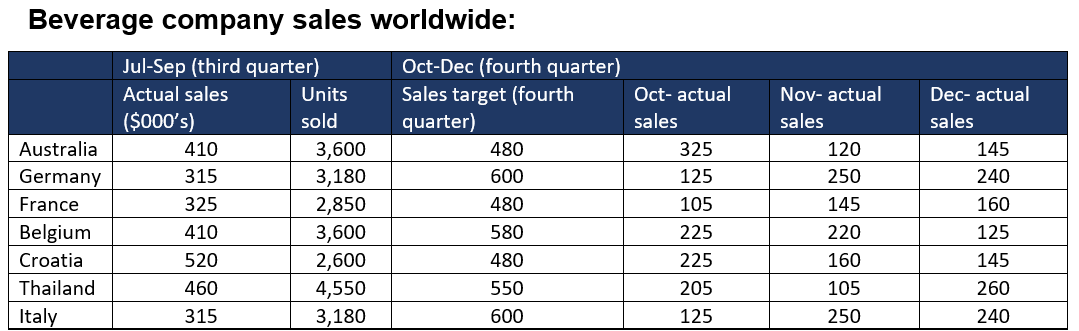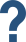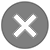Flags

# Free Numerical Reasoning Test and Free Numerical Aptitude Test Practice Online

### What Is a Numerical Reasoning Test or a Numerical Aptitude test in Australia?

Numerical reasoning tests (or numerical aptitude tests) are used to measure your ability to work with and understand numbers, as part of your decision making.

Numerical reasoning test questions are an essential component of cognitive, aptitude and psychometric tests.

Numerical reasoning test questions typically include a wide range of numerical test questions styles, such as; tables & graphs, ratios, percentages and number series. The style of numerical test questions and the level of difficulty is customised to the job you are applying for.

Numerical reasoning test questions offer you a large amount of information to process in a very short time, making these types of questions fairly challenging.

Our expert Psychometricians have developed a list of successful preparation packages to ensure that you pass your numerical reasoning test with flying colours:

1. Real online practice numerical tests customised to your job, with detailed answer explanations.
2. Online numerical test courses.
3. Face-to-face or Skype video tutoring with our expert Psychometricians.

# Numerical Question Styles: Get to know the question style that you will get

Our expert Psychometricians have collected information about 5 styles of numerical reasoning test question that are most commonly used.

It is important to get to know the different styles of numerical reasoning test question so you will be adequately prepared for your numerical reasoning test.

# The style of numerical test question in your test depends on the job and not on the psychometric test developer

Remember that psychometric test developers offer special styles of numerical test question for each job. Don't just practice for one style of numerical test question or for a specific psychometric test developer. You must practice for the style of numerical test question that you will get in your real test. This depends on the job you are applying for.

# Numerical test question's level of difficulty

Each style of numerical test question has levels of difficulty. This depends on the job you are applying for. Make sure that you are preparing for the level of difficulty suitable for the job you are applying for.

# Five Free Numerical Reasoning and Aptitude Practice Tests

Below you will be able to practice short numerical reasoning tests. Each practice test will focus on one style of numerical test question.

We also offer a detailed answer explanation at the end of each practice test. We would strongly advise that you read the detailed answer explanations to learn how to answer similar numerical test questions in your real test.

1.     Tables and Graphs style

This practice numerical reasoning test has a list of questions that measure your numerical reasoning skills by interpreting information offered in tables and graphs.

This free numerical test includes:

XX test questions

XX minutes

Take Free Numerical Reasoning Test – Tables and Graphs2.     Ratios style

This practice numerical reasoning test focuses on measuring your ability to understand the concept of ratios, calculate ratios and use ratios to solve problems.

This free numerical test includes:

XX test questions

XX minutes

Take Free Numerical Reasoning Test – Ratios3.     Percentages style

This practice numerical reasoning test focuses on measuring your ability to understand the concept of percentages, calculate percentages and use percentages to make decisions.

This free numerical test includes:

XX test questions

XX minutesTake Free Numerical Reasoning Test – Percentages

4.     Number series style

This practice numerical aptitude test focuses on measuring your ability to work with numbers and identify patterns in numbers to make decisions.

This free numerical test includes XX test questions and you have XX minutes.

Take Free Numerical Reasoning Test – number series5.  Numbers in buubles

This practice numerical aptitude test focuses on measuring your ability to quickly do basic calculations with numbers.

This free numerical test includes XX test questions and you have XX minutes.

Take Free Numerical Aptitude Test - numbers in bubbles# Job Customised Numerical Reasoning Tests - Practice Test Questions That You Will have in Your Real Test

While most candidates will receive an invite to take a numerical reasoning test, the style of test questions is likely to be very different. The style of numerical test questions is customised to the job you are applying for.

You must practice for the correct style of numerical test questions to avoid wasting your time.

Our expert psychometricians have collected example numerical reasoning test questions with step-by-step explanations:

We have collected some example numerical reasoning test questions of different styles to assist you in understanding what type of questions you are likely to be asked to solve in your real numerical test.

Getting to know the type of numerical test questions and the correct techniques required to solve them is very important to allow you to successfully pass your numerical reasoning test.

Each example numerical reasoning test also has a detailed step-by-step answer explanation.

# “Tables & Graphs” numerical test questions

The aim of numerical reasoning tests is to measure your ability to understand information presented in a numerical form. One of the most popular forms of presenting numerical data is tables and graphs.

In a graph and table style numerical test question, you will be asked to quickly read and interpret information presented in a table or a graph. Your task will be to find the relevant information, then use this information to find the correct answer.

What to expect in a table and graph test question?

• A typical numerical test question in this style will have a table with numerical information presented in many columns and lines or a graph, such as a line or bar graph.
• The table or graph will typically have far more information than you need to answer the question.
• Your task will be to identify the relevant information and use it to answer the test question.

Example numerical reasoning test question - tableWhat is the ratio of the differences in actual sales to target sales in Thailand and Italy during the fourth quarter?

To answer this test question, we need to review the information provided in the table. According to the table, Thailand’s fourth-quarter sales target was 550, but actual sales were: 205 + 105 + 260 = 570. This is a difference of 20 (570 – 550). Italy’s target was 600 sales, but actual sales were: 125 + 250 + 240 = 615. The difference here was 15 (615 – 600). Therefore, the Thailand to Italy ratio of differences is 20:15 = 4:3.

# Example numerical reasoning test question - graph

Getting the right techniques to solve table and graph style numerical test questions, in the quickest way possible, is crucial to your success.

Remember: don't waste time practising numerical test question styles that you will not have in your real numerical reasoning test.

# “Ratio” numerical test questions

Ratios seem to be a very popular style to measure candidates’ numerical reasoning skills. A ratio can be displayed as a straightforward question about a ratio of X to Y or it can be incorporated in an indirect way such as in currency conversions. In the indirect ratios you will always be given ratios so you can use them to calculate or convert the currency or any other unit.

Example for a direct ratio numerical test question:

Example for an indirect ratio style test question:

Remember: don't waste time practising numerical test question styles that you will not have in your real numerical reasoning test.

# “Percentages” numerical test questions

Percentages seem to appear in most, if not all, numerical reasoning tests. Therefore, it is important to ensure that you know how to calculate percentages and what the concept of percentages means.

In a typical percentage numerical test question, you will need to calculate a percentage change (for example the percentage change in sales between two months) or you will be given a percentage increase or decrease of a figure and you will be asked to calculate the final figure (for example a price increase or decrease).

Example numerical reasoning test question - percentages

# “Number series” numerical test questions

Numerical test questions that include number series can be separated into to two groups. The first group is one long sequence of numbers that make up a number series. The second group is a Sudoku type of number series. Typically appearing in a 3 by 3 table.

In the number series questions, the sequence of numbers have logical rules and patterns that make them a group. The logical rules and patterns have to do with the specific position of each number in the sequence.

Your task in solving number series questions is to find the unique patterns and logical rules for each series, then use these patterns and logical rules to find a missing number.

Learning the specific techniques of how to quickly analyse number series is crucial, as you are given a very short amount of time to complete each test question.

Example numerical reasoning test question – line number series

Example numerical reasoning test question – Sudoku number seriesNeed HelpNeed Help